# huangx607087学习格密码的笔记5

## 12.格约简算法

### 12x02 LLL格约简算法

$$\vec v_i^*=v_i-\sum_{j=1}^{i-1}u_{ij}\vec v_j*$$

UPD 2021.2.9：原来这里的公式改成了图片，因为在网页上公式显示异常。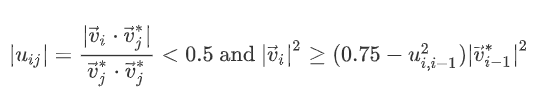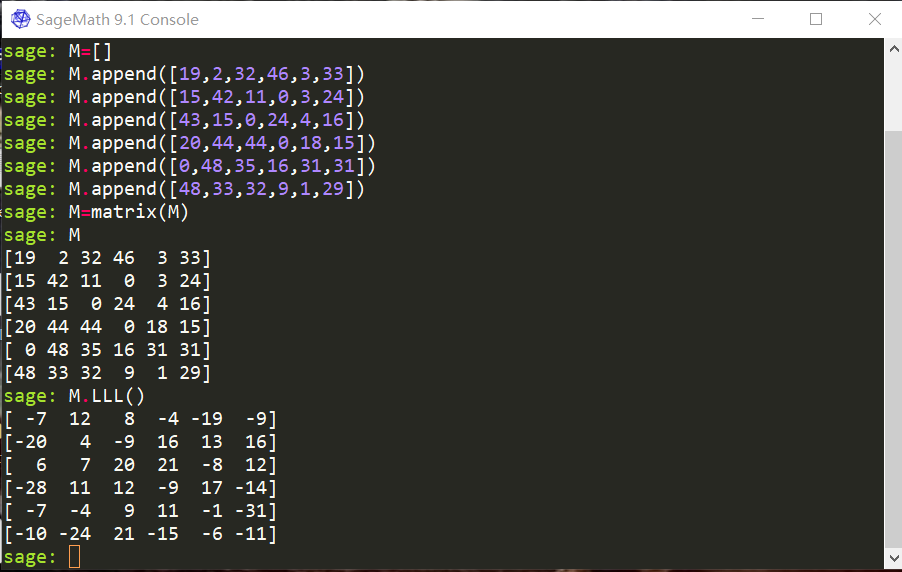## 13.LLL在密码分析中的应用

### 13x02 回顾笔记1中背包密码中的LLL算法破解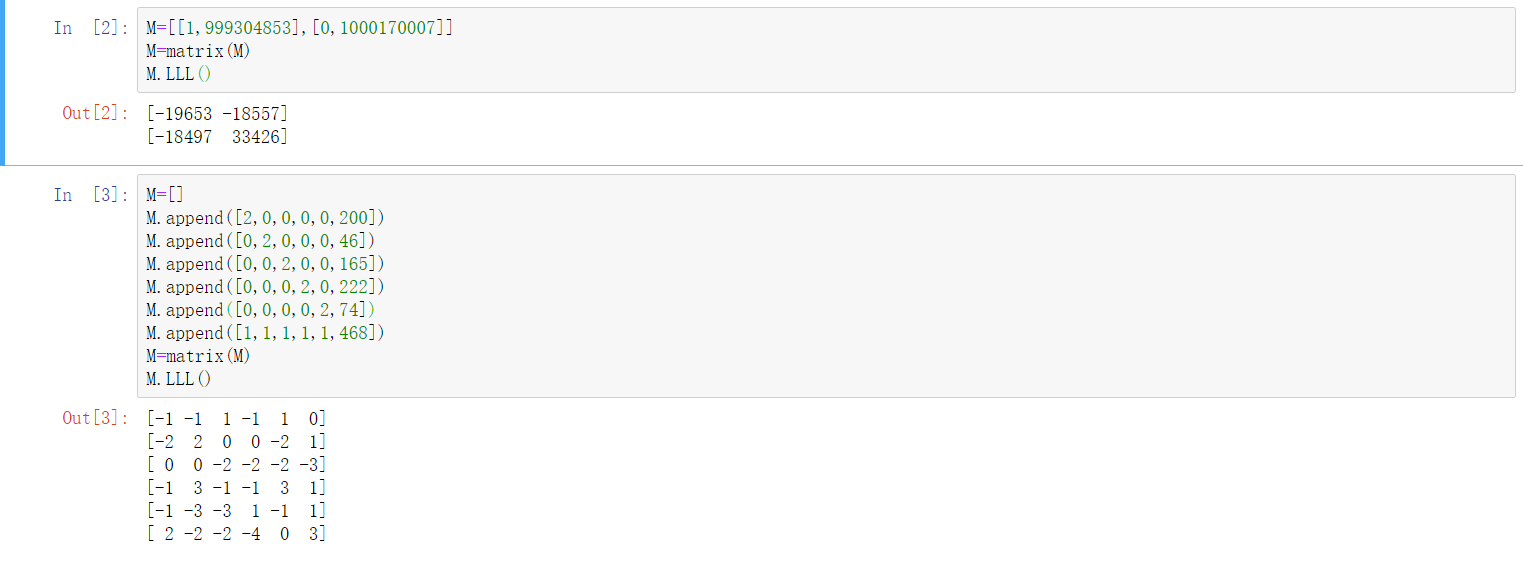### 13x03 回顾笔记3中的GGH公钥密码系统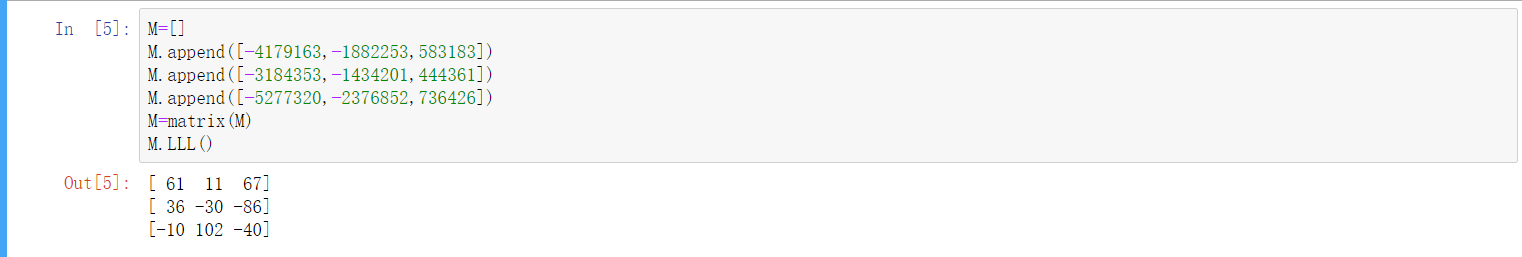$$\vec v=35\vec w_1-86\vec w_2+32\vec w_3=(79081423, 35617459, −11035471)$$

### 13x04 回顾笔记4中的NTRU算法

$$h(x)=20x^6+40x^5+2x^4+38x^3+8x^2+26x+30$$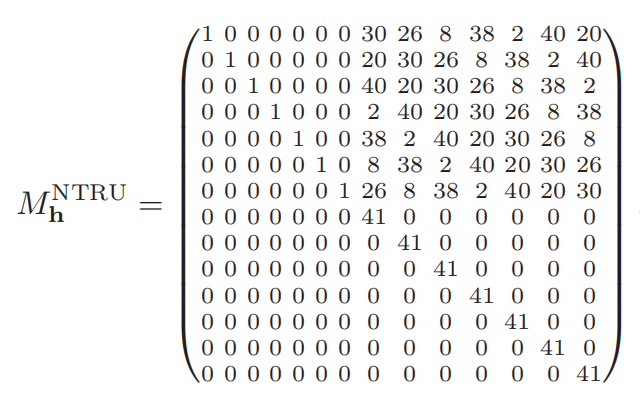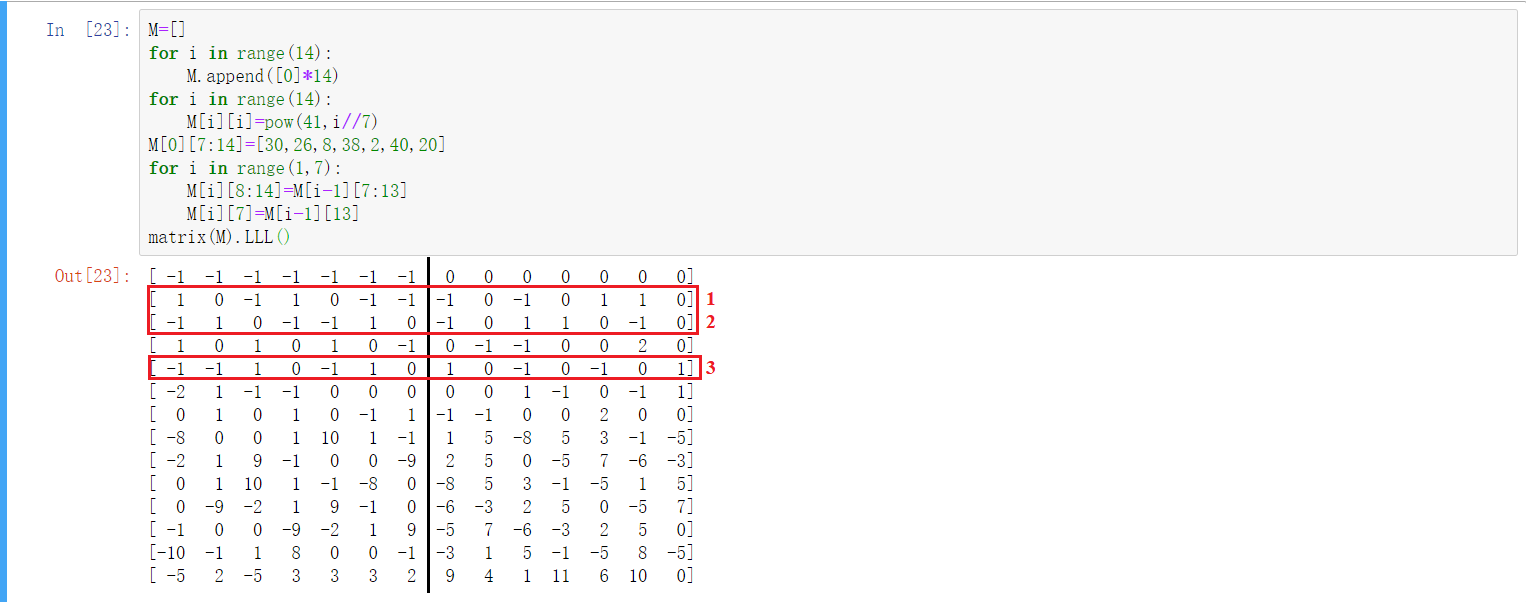## 14.例题选讲[UPDATE 2021.2.20]

### 14x01 NPUCTF2020[BABYLCG]

update 2021.2.8

#### STEP 2 初步分析，确定构建格矩阵的法则

$$c_2+d_2\equiv a(c_1+d_1)+b \pmod m$$

$$c_2+d_2=ac_1+ad_1+b+km$$

$$d_2=ad_1+km+ac_1+b-c_2=f(k,d_1)$$

#### STEP 3 从理论走向实践

$$d_2=ad_1+km+(ac_1+b-c_2)=f(k,d_1)+C$$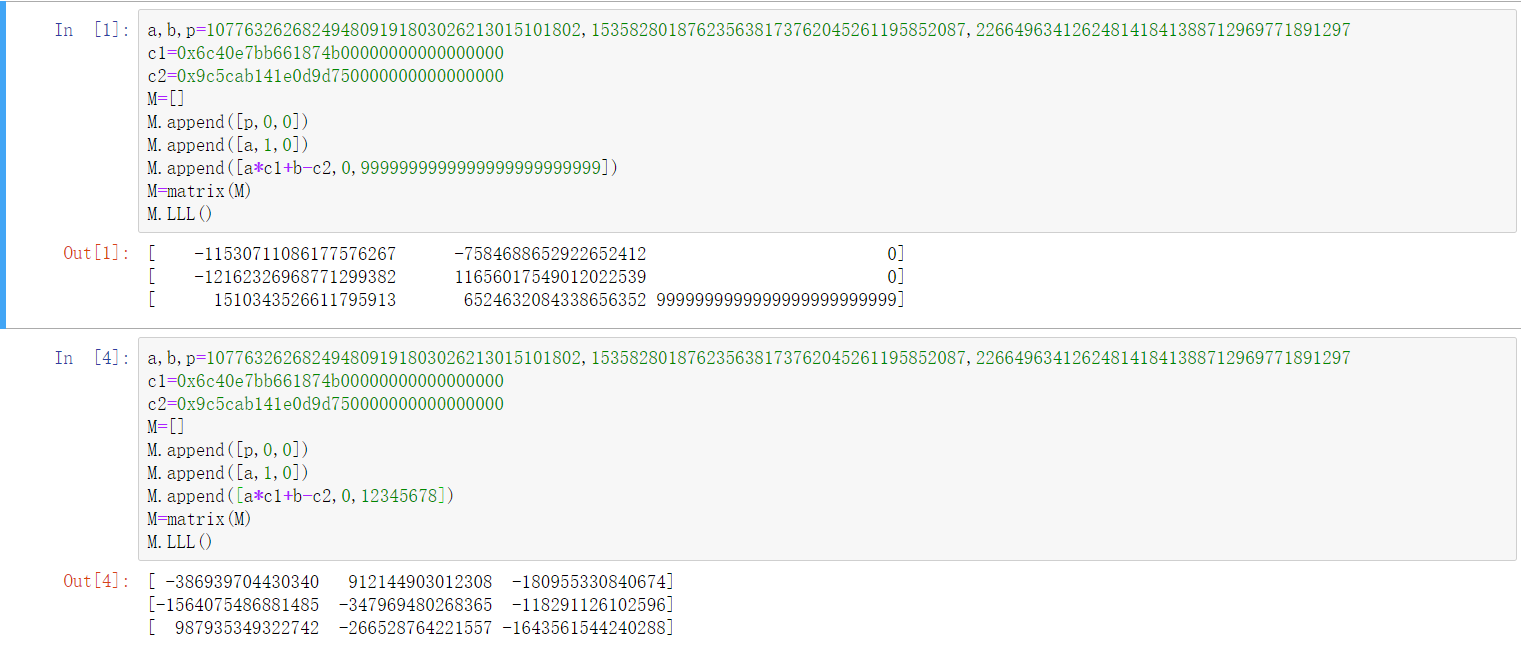### 14x02 ACTF2021 [hashgame]

Updated 2021.2.20

#### Step 2：初步分析，构造矩阵

$$hg^{32}+\sum_{i=1}^{32} x_i g^{33-i}\equiv hg^{32}+\sum_{i=1}^{32} y_i g^{33-i} \pmod{2^{256}}$$

$$\sum_{i=1}^{32} x_i g^{33-i}\equiv\sum_{i=1}^{32} y_i g^{33-i} \pmod{2^{256}}$$

$$\sum_{i=1}^{32} x_i g^{32-i}=\sum_{i=1}^{32} y_i g^{33-i} +2^{256}k$$

$$\sum_{i=1}^{32}(x_i-y_i)g^{33-i}=2^{256}k$$

$$\sum_{i=1}^{32}(x_i-y_i)g^{33-i}-2^{256}k=0$$#### Step 3：构造并调整矩阵

$$a^2+b^2<(a+b)^2$$目录